Function Repository Resource:

# PeriodicBoxDistance

Evaluate the shortest distance between two points in a box with periodic boundary conditions

Contributed by: Matt Kafker
 ResourceFunction["PeriodicBoxDistance"][u,v,L] gives the shortest distance between vectors u and v in a cubic box of side-length L centered on the origin with periodic boundary conditions.

## Details

ResourceFunction["PeriodicBoxDistance"] works for boxes in any number of spatial dimensions.

## Examples

### Basic Examples (3)

Compute a 1D periodic box distance in the "box" represented by the interval [-1,1]:

 In:=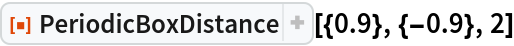Out=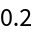PeriodicBoxDistance reverts to Euclidean distance when points are close by within the box:

 In:=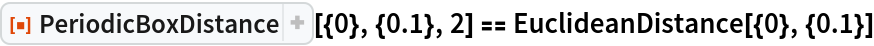Out=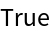Parallel points on the boundaries of the box have zero separation:

 In:=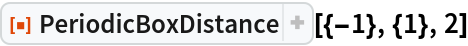Out=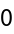### Scope (1)

PeriodicBoxDistance also works in higher dimensions:

 In:=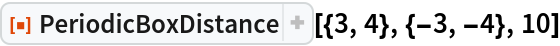Out=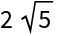### Neat Examples (1)

Compare PeriodicBoxDistance from the origin with Euclidean distance in two dimensions:

 In:=Out=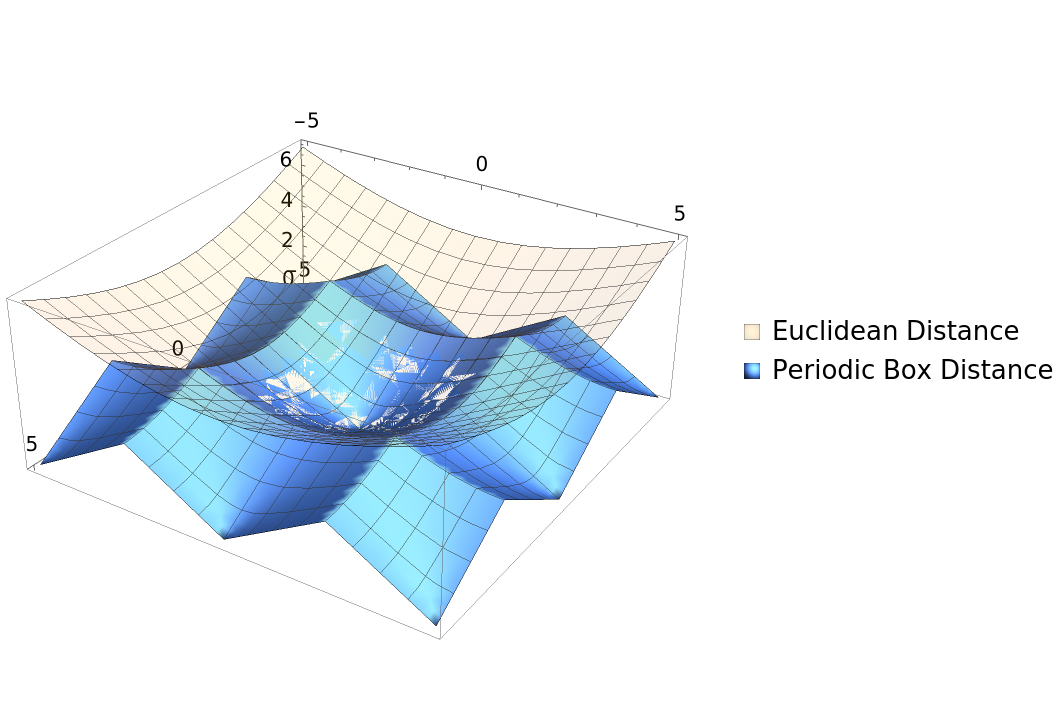## Publisher

Wolfram Summer School

## Version History

• 1.0.0 – 24 August 2021